# ANGULAR MEASUREMENT Angle In Geometry an angle is

• Slides: 21ANGULAR MEASUREMENT Angle: In Geometry an angle is the figure formed by two rays sharing a common end point, called the vertex of the angle. The magnitude of the angle is the "amount of rotation" that separates the two rays, and can be measured by considering the length of circular arc swept out when one ray is rotated about the vertex to coincide with the other 24/02/2012 lec # 17 & 18 1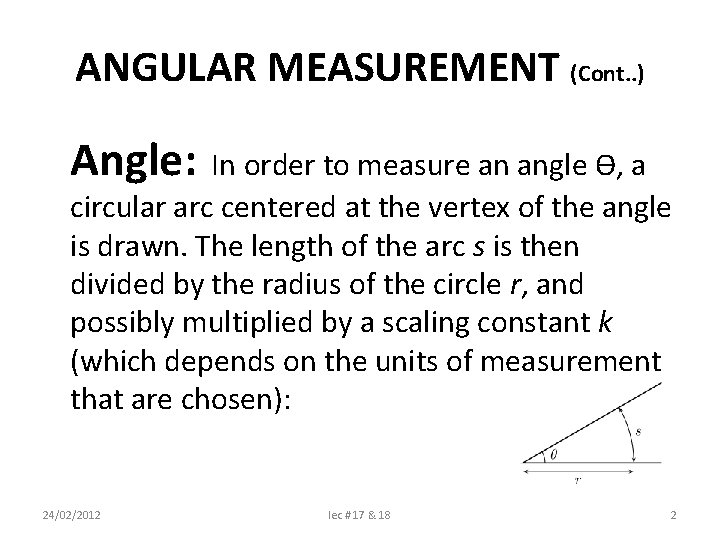ANGULAR MEASUREMENT (Cont. . ) Angle: In order to measure an angle Ɵ, a circular arc centered at the vertex of the angle is drawn. The length of the arc s is then divided by the radius of the circle r, and possibly multiplied by a scaling constant k (which depends on the units of measurement that are chosen): 24/02/2012 lec # 17 & 18 2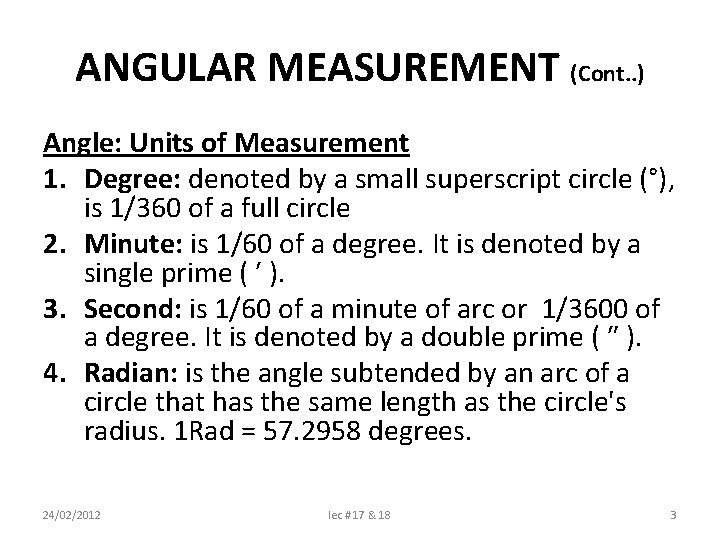ANGULAR MEASUREMENT (Cont. . ) Angle: Units of Measurement 1. Degree: denoted by a small superscript circle (°), is 1/360 of a full circle 2. Minute: is 1/60 of a degree. It is denoted by a single prime ( ′ ). 3. Second: is 1/60 of a minute of arc or 1/3600 of a degree. It is denoted by a double prime ( ″ ). 4. Radian: is the angle subtended by an arc of a circle that has the same length as the circle's radius. 1 Rad = 57. 2958 degrees. 24/02/2012 lec # 17 & 18 3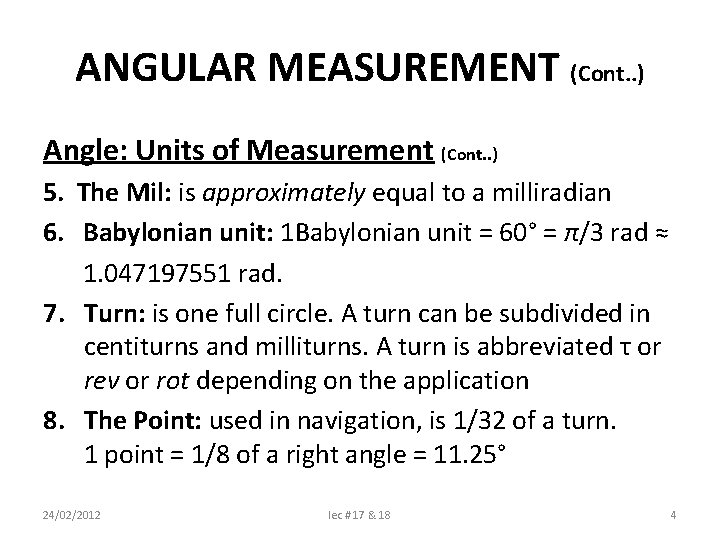ANGULAR MEASUREMENT (Cont. . ) Angle: Units of Measurement (Cont. . ) 5. The Mil: is approximately equal to a milliradian 6. Babylonian unit: 1 Babylonian unit = 60° = π/3 rad ≈ 1. 047197551 rad. 7. Turn: is one full circle. A turn can be subdivided in centiturns and milliturns. A turn is abbreviated τ or rev or rot depending on the application 8. The Point: used in navigation, is 1/32 of a turn. 1 point = 1/8 of a right angle = 11. 25° 24/02/2012 lec # 17 & 18 4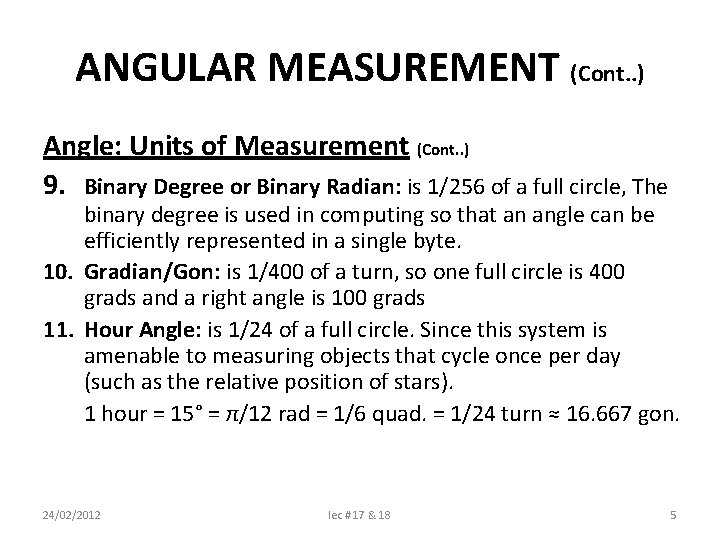ANGULAR MEASUREMENT (Cont. . ) Angle: Units of Measurement (Cont. . ) 9. Binary Degree or Binary Radian: is 1/256 of a full circle, The binary degree is used in computing so that an angle can be efficiently represented in a single byte. 10. Gradian/Gon: is 1/400 of a turn, so one full circle is 400 grads and a right angle is 100 grads 11. Hour Angle: is 1/24 of a full circle. Since this system is amenable to measuring objects that cycle once per day (such as the relative position of stars). 1 hour = 15° = π/12 rad = 1/6 quad. = 1/24 turn ≈ 16. 667 gon. 24/02/2012 lec # 17 & 18 5INSTRUMENTS FOR ANGULAR MEASUREMENT 1. Pratractors: 2. 3. 4. 5. 6. 7. 8. 9. a. b. c. Vernier Bevel Protractor Dial Bevel Protractor Optical Bevel Protractor Sine Bars Sine tables Sine Centre Angle Gauges Sprit Level Clinometers Plain Index Centers Optical Instruments for angular measurement such as autoclinometer 24/02/2012 lec # 17 & 18 6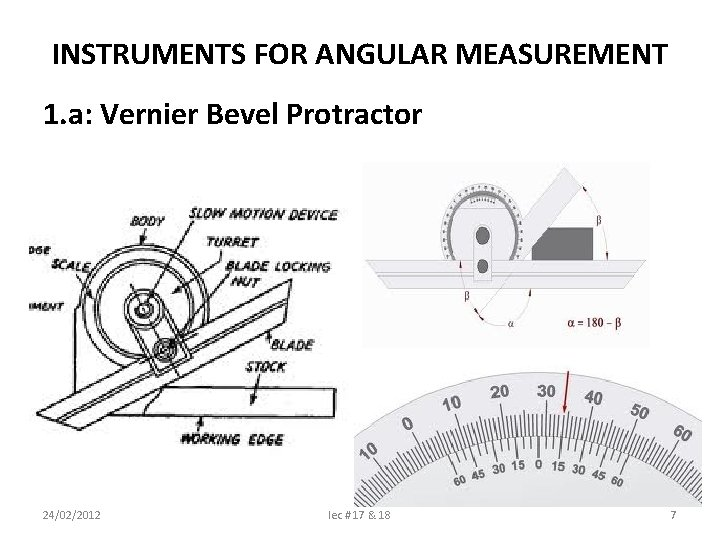INSTRUMENTS FOR ANGULAR MEASUREMENT 1. a: Vernier Bevel Protractor 24/02/2012 lec # 17 & 18 7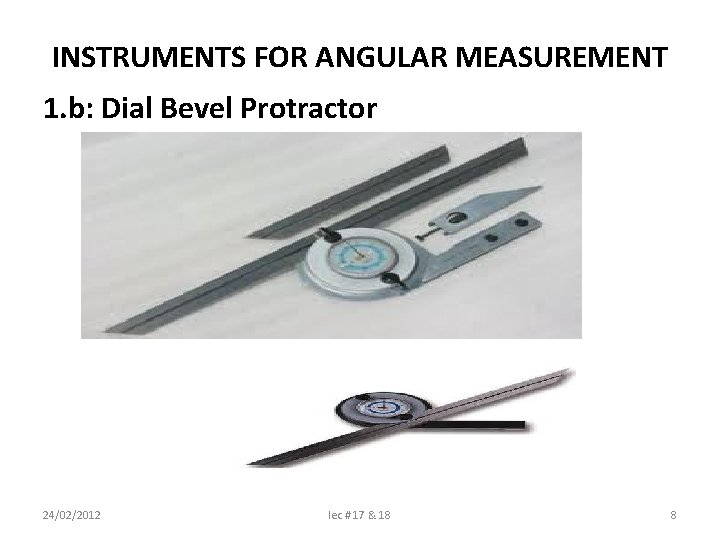INSTRUMENTS FOR ANGULAR MEASUREMENT 1. b: Dial Bevel Protractor 24/02/2012 lec # 17 & 18 8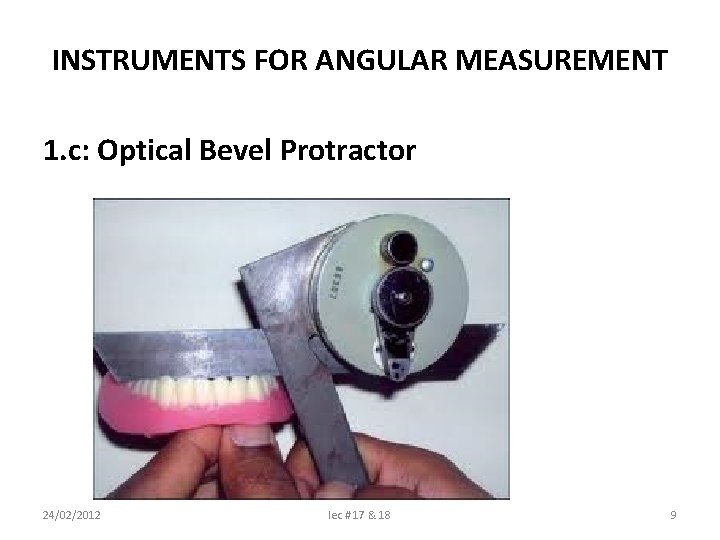INSTRUMENTS FOR ANGULAR MEASUREMENT 1. c: Optical Bevel Protractor 24/02/2012 lec # 17 & 18 9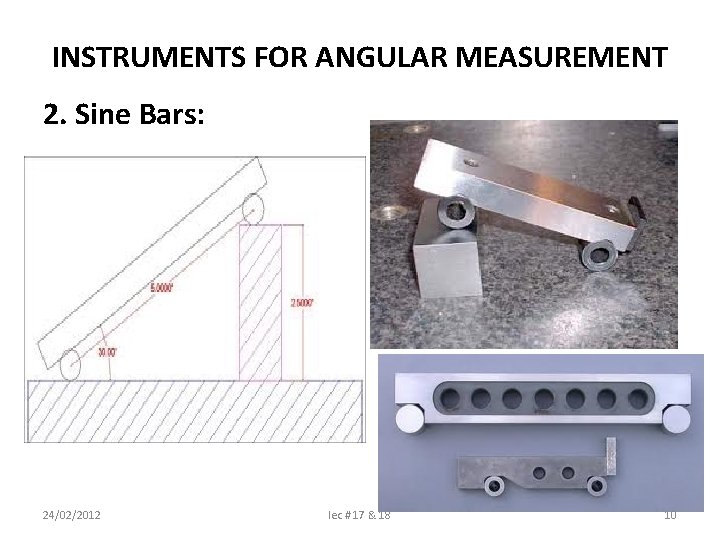INSTRUMENTS FOR ANGULAR MEASUREMENT 2. Sine Bars: 24/02/2012 lec # 17 & 18 10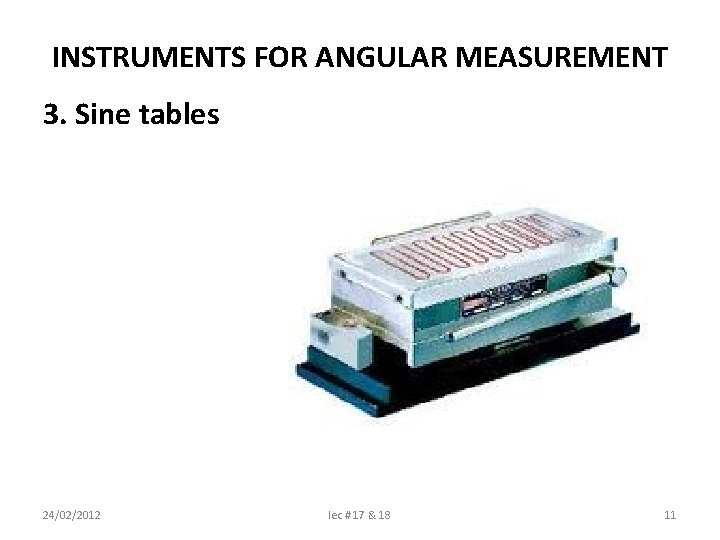INSTRUMENTS FOR ANGULAR MEASUREMENT 3. Sine tables 24/02/2012 lec # 17 & 18 11INSTRUMENTS FOR ANGULAR MEASUREMENT 4. Sine Centre: 24/02/2012 lec # 17 & 18 12INSTRUMENTS FOR ANGULAR MEASUREMENT 5. Angle Gauges: 24/02/2012 lec # 17 & 18 13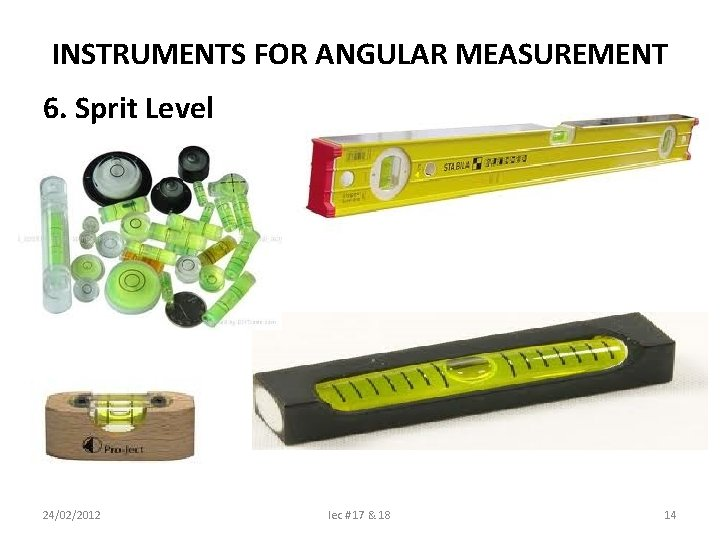INSTRUMENTS FOR ANGULAR MEASUREMENT 6. Sprit Level 24/02/2012 lec # 17 & 18 14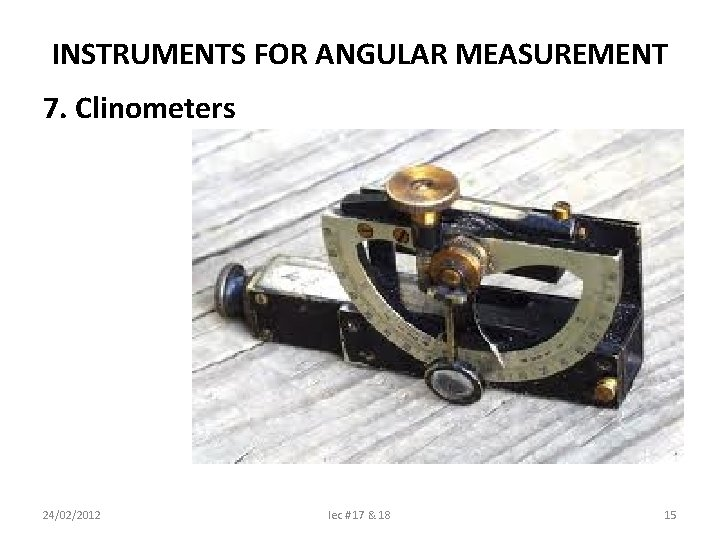INSTRUMENTS FOR ANGULAR MEASUREMENT 7. Clinometers 24/02/2012 lec # 17 & 18 15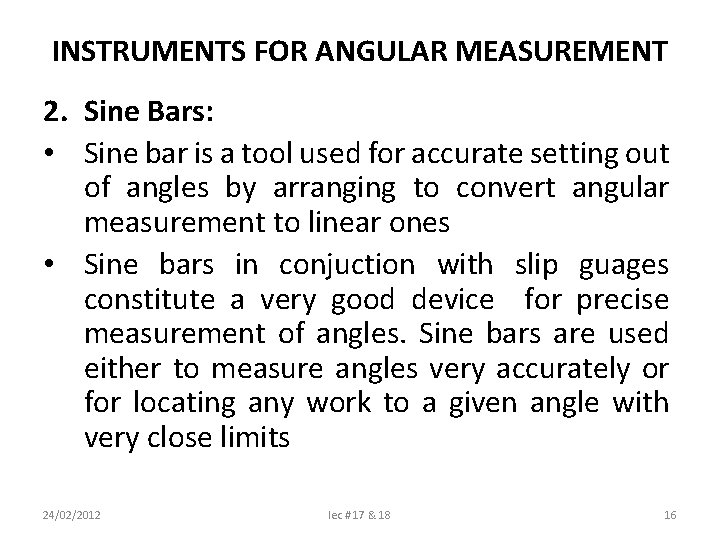INSTRUMENTS FOR ANGULAR MEASUREMENT 2. Sine Bars: • Sine bar is a tool used for accurate setting out of angles by arranging to convert angular measurement to linear ones • Sine bars in conjuction with slip guages constitute a very good device for precise measurement of angles. Sine bars are used either to measure angles very accurately or for locating any work to a given angle with very close limits 24/02/2012 lec # 17 & 18 16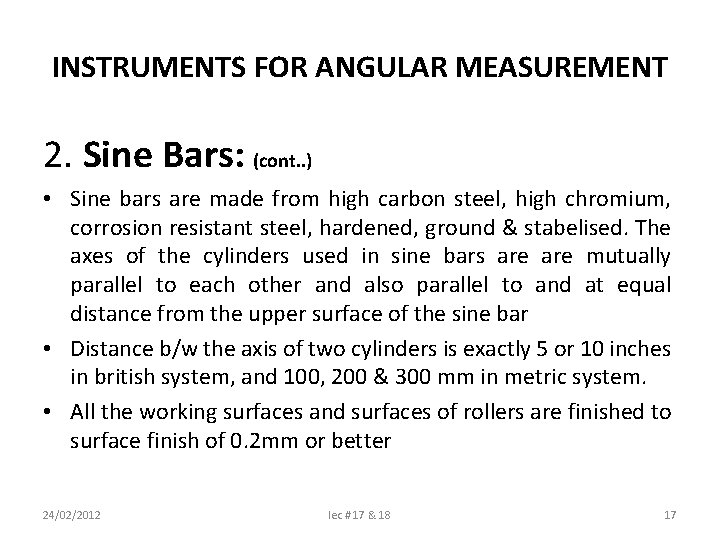INSTRUMENTS FOR ANGULAR MEASUREMENT 2. Sine Bars: (cont. . ) • Sine bars are made from high carbon steel, high chromium, corrosion resistant steel, hardened, ground & stabelised. The axes of the cylinders used in sine bars are mutually parallel to each other and also parallel to and at equal distance from the upper surface of the sine bar • Distance b/w the axis of two cylinders is exactly 5 or 10 inches in british system, and 100, 200 & 300 mm in metric system. • All the working surfaces and surfaces of rollers are finished to surface finish of 0. 2 mm or better 24/02/2012 lec # 17 & 18 17INSTRUMENTS FOR ANGULAR MEASUREMENT 2. Sine Bars: (cont. . ) Measures for Accurate Results: i. Contact rollers must be equal diameter & true geometric cylinders ii. Distance b/w the rollers axis must be precise & known, & these axis must be mutually parallel iii. The upper surface of the bar must be flat & parallel with the roller axes, & equidance from each other 24/02/2012 lec # 17 & 18 18INSTRUMENTS FOR ANGULAR MEASUREMENT 2. Sine Bars: (cont. . ) Use of Sine Bars 1. Measuring known angles, or locating any work to a given angle 2. Checking of unknown angles 3. Checking of Unknown angles of heavy components 24/02/2012 lec # 17 & 18 19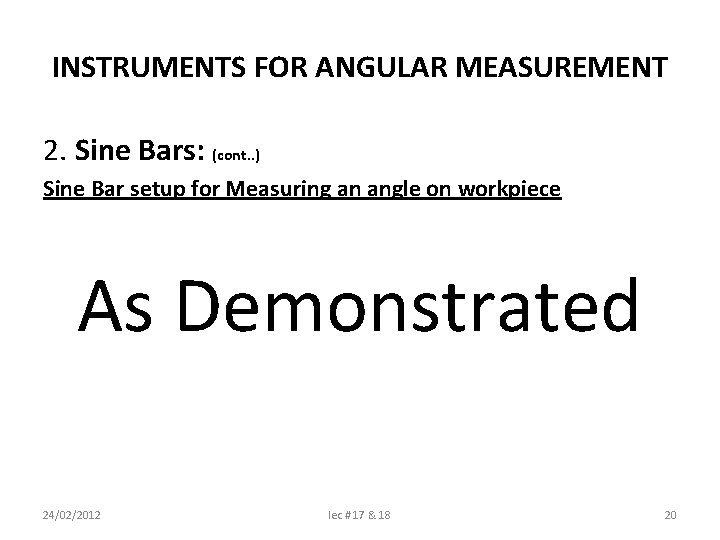INSTRUMENTS FOR ANGULAR MEASUREMENT 2. Sine Bars: (cont. . ) Sine Bar setup for Measuring an angle on workpiece As Demonstrated 24/02/2012 lec # 17 & 18 20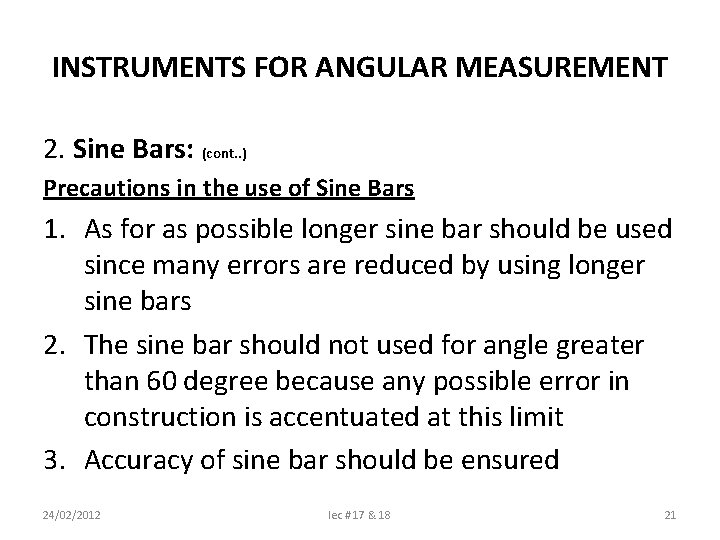INSTRUMENTS FOR ANGULAR MEASUREMENT 2. Sine Bars: (cont. . ) Precautions in the use of Sine Bars 1. As for as possible longer sine bar should be used since many errors are reduced by using longer sine bars 2. The sine bar should not used for angle greater than 60 degree because any possible error in construction is accentuated at this limit 3. Accuracy of sine bar should be ensured 24/02/2012 lec # 17 & 18 21